# SSC CGL 2018 Practice Test Papers | Quantitative Aptitude (Day-61)

Dear Aspirants, Here we have given the Important SSC CGL Exam 2018 Practice Test Papers. Candidates those who are preparing for SSC CGL 2018 can practice these questions to get more confidence to Crack SSC CGL 2018 Examination.

[WpProQuiz 3776]

Click “Start Quiz” to attend these Questions and view Explanation

1) P can do a piece of work in 12 days and K can do the same job in 15 days. If K will leave three days before the work is completed, then how many days P and K work together?

a) 3 days

b) 5 days

c) 10 days

d) 6 days

2) An article is marked Rs. 2500 and sold it on Rs.2250. What is the reduction percentage as discount?

a) 8 %

b) 4 %

c) 10 %

d) 20 %

3) Average of 40 observations is 16. If 2 is subtracted from each observation, then what will be the new Average of the observation?

a) 16

b) 18

c) 15

d) 14

4) A train can travel 25% faster than a car. Both start from point A at the same time and reach point B 165 km away at the same time. On the way the train takes 40 minutes for stopping at the stations. What is the difference in the speeds (in km/hr) of train and car?

a) 15. 75

b) 12. 375

c) 10.375

d) 11. 75

5) If 3A = 2B and 2B = 3C, then what is A: B: C?

a) 1: 2: 3

b) 3: 2: 1

c) 2: 3: 2

d) 3: 2: 3

6) In the following question, select the missing number from the given series.

15, 17, 20, 22, 25, 27, 30, 32, ?

a) 34

b) 36

c) 30

d) 35

7)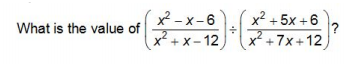a) 0

b) 1

c) (x + 2) (x+3)

d) (x + 2) / (x + 3)

8) How many numbers are there from 300 t0 500 which are divisible by both 4 and 7?

a) 6

b) 7

c) 8

d) 10

9) Which is the largest among the numbers √5, 3√7 and 4√13?

a) √5

b) 3√7

c) 4√13

d) None of these

10) What is the sum of first 30 even numbers?

a) 900

b) 930

c) 960

d) 990

P – 1/12 days

K – 1/15 days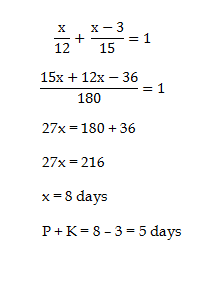M.P = 2500

1. P = 2250

Discount amount = 250

Discount % = 250 * 100 / 2500 = 10%

Total of Observation = 40 * 16 = 640

Total of new observation = 560 – (40*2) = 640 – 80 = 560

New average = 560 / 40 = 14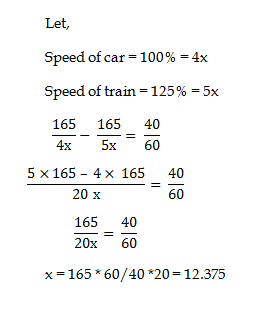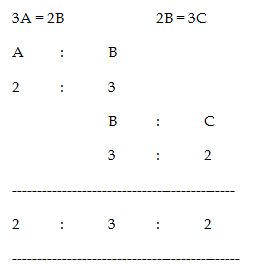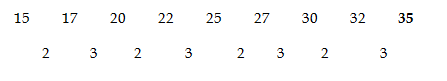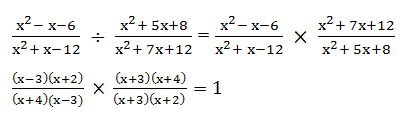300 ÷ (7 × 4) = 7

500 ÷ (7 × 4) = 17

Totally 10 number are there from 300 to 500 which are divisible by both 4 and 7

√5 = 2.236 < 3√7 = 7.932 < 4√13 = 14. 422

Sum of first n even integers = n (n + 1) = 30 * 31 = 930